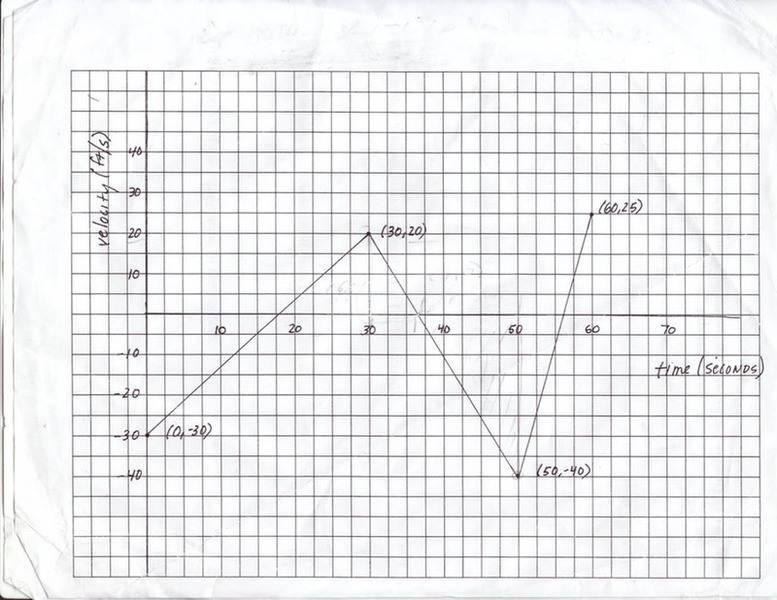# What Is the X Coordinate of the Particle?

1. The problem statement, all variables and given/known data
The graph below represents the time versus velocity of a particle. The x coordinate of the particle at 30 seconds is 20 feet.

What is the x coordinate of the particle at 50 seconds?

2. Relevant equations
These are the equations I think could be used to solve this problem; the class hadn't been exposed to much else at this point.

Eq. 1 v = v0 + at

Eq. 2 x - x0 = v0t + 1/2at2

Eq. 3 v2 = v20 + 2a(x - x0)

Eq. 4 x - x0 = 1/2(v0 + v)t

Eq. 5 x - x0 = vt + 1/2at2

3. The attempt at a solution
The teacher told us that the answer to this problem is -180 but definitely NOT -40.

My method went like this:

I assumed when the problem asked me for the x coordinate, it meant x as in position, but not x as in the x and y axis on the graph below. From that, I set out to solve this problem using equation 2 and setting it up for final position like so:

x = v0t + 1/2at2 + x0

then since I knew I didn't have the acceleration for this problem, I tried to find it:

a = -40 - 20 / 50 - 30 = -3ft/s

once I got the acceleration of -3ft/s I added my known values to the equation I setup:

x = 20*50 + (1/2*-3)502 + 20

and then I got:

x = 1000 + (-3750) + 20 = -2730

I've honestly tried ALOT of other ways including trying to calculate the acceleration of the first point on the graph and then substituting that for my a and that gave me weird answers also.#### collinsmark

Homework Helper
Gold Member
I assumed when the problem asked me for the x coordinate, it meant x as in position, but not x as in the x and y axis on the graph below.
Yes, that's right.The x-component of the graph below is time. But the "x" that you are looking for is distance. So you are right.From that, I set out to solve this problem using equation 2 and setting it up for final position like so:

x = v0t + 1/2at2 + x0
Just make sure you are careful about how to interpret t in your equation. You are starting at t0 = 30 sec, not zero seconds. So everything needs to be done with respect to t0 = 30 sec as a starting point.
then since I knew I didn't have the acceleration for this problem, I tried to find it:

a = -40 - 20 / 50 - 30 = -3ft/s
'Looks good to me!once I got the acceleration of -3ft/s I added my known values to the equation I setup:

x = 20*50 + (1/2*-3)502 + 20
Try it again, but this time be more careful about how you interpret t. The conditions x0 = 20 ft, and v0 = 20 ft/sec are true when t = 30 sec (not when t = 0 sec). You need to subtract off 30 sec from t to make sure everything lines up. In other words,

x = x0 + v0(t - 30) + (1/2)a(t - 30)2

Dude...WOW. I can't believe after countless hours all I needed to do was calculate the change in time. Thank you SO much!

As a clarification though, whenever I see t in a formula, does that mean I'm always supposed to find t - t0? I never thought to do that because nothing I read up to this point seemed to suggest I should, that is unless I was trying to calculate the change in t as part of the formula to find average speed or average acceleration.

#### collinsmark

Homework Helper
Gold Member
Dude...WOW. I can't believe after countless hours all I needed to do was calculate the change in time. Thank you SO much!

As a clarification though, whenever I see t in a formula, does that mean I'm always supposed to find t - t0? I never thought to do that because nothing I read up to this point seemed to suggest I should, that is unless I was trying to calculate the change in t as part of the formula to find average speed or average acceleration.
Essentially, yes. One way to write that particular kinematics equation is

x = x0 + v0t) + (1/2)at)2,

which is another way of saying,

x = x0 + v0(t - t0) + (1/2)a(t - t0)2

[Edit: Just make sure you understand what t0 is. The constant t0 is the specific time, within the interval of uniform acceleration, that that x = x0 and v = v0.]

Last edited:

### The Physics Forums Way

We Value Quality
• Topics based on mainstream science
• Proper English grammar and spelling
We Value Civility
• Positive and compassionate attitudes
• Patience while debating
We Value Productivity
• Disciplined to remain on-topic
• Recognition of own weaknesses
• Solo and co-op problem solving